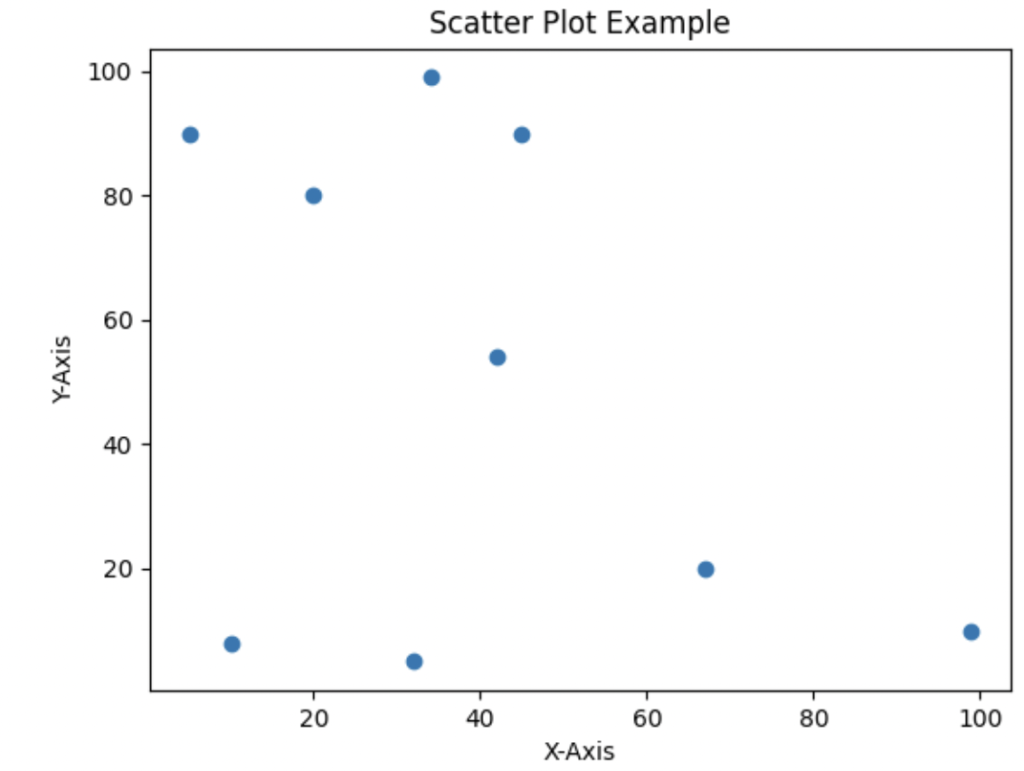# Matplotlib savefig: The Complete Guide

0
17Matplotlib is a highly useful visualization library for graphs and charts in Python. It is a multi-platform data visualization library built on NumPy arrays and designed to work with the broader library.

## Matplotlib savefig

Matplotlib.pyplot.savefig() is a library function used to save the created figure. The matplotlib.savefig() function saves the figure in the image format.

### Syntax

``````matplotlib.pyplot.savefig(fname, dpi='figure', format=None, metadata=None, bbox_inches=None,
pad_inches=0.1, facecolor='auto', edgecolor='auto', backend=None, **kwargs) ``````

### Arguments

The matplotlib.pyplot.savefig() function has one required argument as a parameter:

1. fname: The fname argument takes a string value as value to this argument. This is the required argument. This argument takes the filename as a value. The file name in which the image is stored is taken as the value.
2. dpi: The dpi stands for dots per inch. This argument takes floating numbers as values. This argument describes the resolution in which the image is created.
3. format: The format takes a string value as a value. This argument describes the file format as value. The format in which the file is stored is passed in this argument. Some of the formats are png, pdf, SVG, etc.
4. metadata: The metadata argument takes a dictionary as value to this argument. The key-value pair is passed in this argument.
5. bbox_inches: This argument takes string values. The given portion of the image is only saved.
6. pad_inches: The amount of padding around the image is passed in this argument. This takes a floating-point number as a value.
7. facecolor: The facecolor of the image is given in this argument.
8. edgecolor: The edge color of the image is given in this argument.
9. backend: String value is taken as value to this argument. The backend is given in this argument.
10. orientation: The orientation in which the image is to be saved is given in this argument. There are two types of orientation one is the portrait, and the other is the landscape.
11. papertype: The type of paper is given in this argument. Some types of papers are letters, legal, executive, etc.
12. transparent: This is a Boolean value. If True, then the axes patches are transparent.
13. bbox_extra_artists: The list of extra artists is passed in this argument. This argument takes a list as value to this argument.
14. pil_kwargs: The additional arguments passed to the image are passed in this argument. This argument accepts dictionary value as value to this function.

### Return value

The savefig() function saves the graphs or charts to an image in the specified location.

### Program for saving a scatter plot using matplotlib.pyplot.savefig

``````# Importing matplotlib.pyplot as plt.
import matplotlib.pyplot as plt

# Importing numpy as np
import numpy as np

# x coordinates are created
x = np.array([5,20,10,67,99,45,32,34,42])

# y coordinates are created
y = np.array([90,80,8,20,10,90,5,99,54])

# scatter plot is created
plt.scatter(x,y)

# x axis is labeled as X-Axis
plt.xlabel('X-Axis')

# y axis is labeled as Y-Axis
plt.ylabel('Y-Axis')

# Title is kept for the Scatter plot
plt.title('Scatter Plot Example')

# Saving the created graph using the savefig function
plt.savefig("Scatter.png")``````

#### OutputIn this program, we imported matplotlib.pyplot for plotting the scatterplot and storing the created scatter plot.

The matplotlib library consists of all the functions for plotting different types of graphs and charts. Then, we imported the numpy library for creating x coordinates and y coordinates. Then, we passed these two coordinates into the scatter function.

The savefig() function creates a scatter plot by combining x and y coordinates. The scatter plot is used when the data points are scattered unordered. In this example, the data points are unordered; hence this example describes the scatter plot properly.

Then, we saved the created scatterplot using the savefig() function. We passed the file name as the argument. We gave Scatter.png as a file name. The savefig() function saves the image in the program file location. We can open the Scatter.png file.

### Program for saving a bar chart using matplotlib.pyplot.savefig

``````# Importing matplotlib.pyplot as plt.
import matplotlib.pyplot as plt

# Importing numpy as np
import numpy as np

# creating x coordinates
x = np.array(["Maths", "Science", "Social", "Computer science"])

# Creating height coordinates
height = np.array([90, 60, 70, 98])

# Creating bar chart using bar function
plt.bar(x, height)

# Saving the created graph using the savefig function
plt.savefig("bar.png",
bbox_inches="tight",
transparent=True,
facecolor="y",
edgecolor='w',
orientation='landscape')``````

#### OutputIn this program, we imported matplotlib.pyplot for plotting the bar chart and for saving the created chart.

The matplotlib library consists of all the functions for plotting different types of graphs and charts. Then we have imported numpy for creating x coordinates. This x array consists of subject names.

Then, we created a y-array. The numpy array consists of subject marks. These two arrays are passed into the bar function. This x array is plotted across the x-axis, and the marks are plotted across the y-axis.

This bar function plots a bar chart across subject and subject marks. Then we have saved the created bar chart using the savefig function. We passed the file name as bar.png, then the bbox_inches as tight, then the face color as yellow, and the edge color as white. Finally, we saved the image in landscape orientation. This image is stored in the working python file location.

That’s it for this tutorial.

## Related posts

Matplotlib legend

Matplotlib histogram

Matplotlib legend

This site uses Akismet to reduce spam. Learn how your comment data is processed.